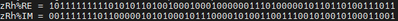Intel® Fortran Compiler
Build applications that can scale for the future with optimized code designed for Intel® Xeon® and compatible processors.
Announcements
27548 Discussions

## ifort doesn't get the same result as gfortran, how can i deal with this.Novice
422 Views

I have a program that I get different results using ifort .vs. gfortran.

The program is:

``````program test

COMPLEX(8) :: z1
REAL(8)  :: cos_i, tmp1, tmp2
COMPLEX(8) :: zRh

cos_i=b'0011111111100101001111001011111010101101101111100101001011101111'
tmp1=b'0100000000011001101111010011101000100111101111000011101001011100'
tmp2=b'1100000000000110011000011000001100010101111100001101100011101100'
z1=cmplx(tmp1, tmp2, 8)

zRh = (cos_i  -z1) / (cos_i  +z1)

102 FORMAT(A, b64.64)
print 102, 'zRh%RE = ', real(zRh)
print 102, 'zRh%IM = ', aimag(zRh)

end program test``````

The compile parameter is：

``````ifort -O0 -fp-model precise -no-ftz

gfortran -O0 ``````

The result of ifort is:The result of gfortran is:I guess ifort has done some optimization.

Can I fix this by increasing compile options?

Labels (1)
• ### Fortran Language

1 SolutionNovice
87 Views

Really， It is more likely a difference in math libraries.

After modifying complex number calculations to real number calculations, gfortran and ifort can get the same calculation results.

Using this code, the result of ifort is the same as before, and the result of gfortran becomes the same as ifort.

``````program test

COMPLEX(8) :: z1
REAL(8)  :: cos_i
COMPLEX(8) :: zRh

cos_i=b'0011111111100101001111001011111010101101101111100101001011101111'
z1%RE=b'0100000000011001101111010011101000100111101111000011101001011100'
z1%IM=b'1100000000000110011000011000001100010101111100001101100011101100'

minus = (cos_i  -z1)

!zRh = (cos_i  -z1) / (cos_i  +z1)

!zRh%RE = ((cos_i-z1%RE)*(cos_i+z1%RE) + (-z1%IM)*(z1%IM)) / ((cos_i+z1%RE) * (cos_i+z1%RE) + (z1%IM)*(z1%IM))
!zRh%IM = ((-z1%IM)*(cos_i+z1%RE) - (cos_i-z1%RE)*(z1%IM)) / ((cos_i+z1%RE) * (cos_i+z1%RE) + (z1%IM)*(z1%IM))

102 FORMAT(A, b64.64)
print 102, 'zRh%RE = ', zRh%RE
print 102, 'zRh%IM = ', zRh%IM

202 FORMAT(A, 1PD27.20)
print 202, 'zRh%RE = ', zRh%RE
print 202, 'zRh%IM = ', zRh%IM

contains

!a/b
function complex_double_div(a, b) result(res)
COMPLEX(8) :: a, b, res

res%RE = (a%RE*b%RE + a%IM*b%IM) / (b%RE*b%RE + b%IM*b%IM)
res%IM = (a%IM*b%RE - a%RE*b%IM) / (b%RE*b%RE + b%IM*b%IM)

return
end function complex_double_div
end program test``````

In addition, I also found that the sqrt calculation results of complex numbers are also different.

The function call can be found from the symbol table.Division may not have such an obvious function call.

7 RepliesBlack Belt Retired Employee
387 Views

You're seeing a single LSB difference between the two. It is more likely a difference in math libraries. It is not reasonable to expect last-bit same results between two different compilers and libraries. See Improving Numerical Reproducibility in C/C++/Fortran (supercomputing.org) for more.Novice
359 Views

I tried these options：

-fimf-precision

-fimf-arch-consistency

The result of the calculation has not changed.Black Belt
382 Views

I suggest you look at the disassembly code of line 12 for each compiler.

And, while you are at it, look at the binary (or hex) values for cos_i and z1.

Could you paste the assembly code and values for cos_i and z1.

Jim DempseyNovice
357 Views

I print the values ​​of cos_i and z1 and the assembly code is generated. Please see the reply below.Novice
357 Views

The new test code:

``````program test

COMPLEX(8) :: z1
REAL(8)  :: cos_i, tmp1, tmp2
COMPLEX(8) :: zRh

cos_i=b'0011111111100101001111001011111010101101101111100101001011101111'
tmp1=b'0100000000011001101111010011101000100111101111000011101001011100'
tmp2=b'1100000000000110011000011000001100010101111100001101100011101100'
z1=cmplx(tmp1, tmp2, 8)
print 102, 'cos_i = ', cos_i
print 102, 'z1%RE = ', real(z1)
print 102, 'z1%IM = ', aimag(z1)

zRh = (cos_i  -z1) / (cos_i  +z1)

102 FORMAT(A, b64.64)
print 102, 'zRh%RE = ', real(zRh)
print 102, 'zRh%IM = ', aimag(zRh)

end program test``````

The result is:The command to generate assembly code is：I don't know much about assembly code.

can you help me, where is the reason for the difference?Black Belt
326 Views

It appears that:

a) the gfortran code is generating SSE code

b) the options chosen for ifort (-fp-model precise) is generating FPU instructions.

Note, the FPU, internally computes intermediary results with 80-bit temporaries whereas SSE does not.

Therefore, the FPU code may produce results with smaller roundoff errors.

Try removing "-fp-model precise" and replacing it with

-arch AVX   (or replace AVX with appropriate architecture for your systems)

or

-xHost      (the architecture of the host at compile time)

Jim DempseyNovice
88 Views

Really， It is more likely a difference in math libraries.

After modifying complex number calculations to real number calculations, gfortran and ifort can get the same calculation results.

Using this code, the result of ifort is the same as before, and the result of gfortran becomes the same as ifort.

``````program test

COMPLEX(8) :: z1
REAL(8)  :: cos_i
COMPLEX(8) :: zRh

cos_i=b'0011111111100101001111001011111010101101101111100101001011101111'
z1%RE=b'0100000000011001101111010011101000100111101111000011101001011100'
z1%IM=b'1100000000000110011000011000001100010101111100001101100011101100'

minus = (cos_i  -z1)

!zRh = (cos_i  -z1) / (cos_i  +z1)

!zRh%RE = ((cos_i-z1%RE)*(cos_i+z1%RE) + (-z1%IM)*(z1%IM)) / ((cos_i+z1%RE) * (cos_i+z1%RE) + (z1%IM)*(z1%IM))
!zRh%IM = ((-z1%IM)*(cos_i+z1%RE) - (cos_i-z1%RE)*(z1%IM)) / ((cos_i+z1%RE) * (cos_i+z1%RE) + (z1%IM)*(z1%IM))

102 FORMAT(A, b64.64)
print 102, 'zRh%RE = ', zRh%RE
print 102, 'zRh%IM = ', zRh%IM

202 FORMAT(A, 1PD27.20)
print 202, 'zRh%RE = ', zRh%RE
print 202, 'zRh%IM = ', zRh%IM

contains

!a/b
function complex_double_div(a, b) result(res)
COMPLEX(8) :: a, b, res

res%RE = (a%RE*b%RE + a%IM*b%IM) / (b%RE*b%RE + b%IM*b%IM)
res%IM = (a%IM*b%RE - a%RE*b%IM) / (b%RE*b%RE + b%IM*b%IM)

return
end function complex_double_div
end program test``````

In addition, I also found that the sqrt calculation results of complex numbers are also different.

The function call can be found from the symbol table.Division may not have such an obvious function call.